Next: B.2 Rectangular Patterns Up: B. Analytical Fourier Transformations Previous: B. Analytical Fourier Transformations

# B.1 Triangular Patterns

An arbitrary triangle consisting of three points {(x1, y1),(x2, y2),(x3, y3)} in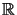2 can always be transformed to the unit triangle {(0, 0),(1, 0),(0, 1)} by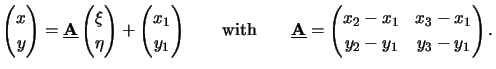(B.2)

The module of the determinant of the transformation matrixequals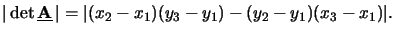(B.3)

Using (B.2), the integral (B.1) writes for triangular patterns to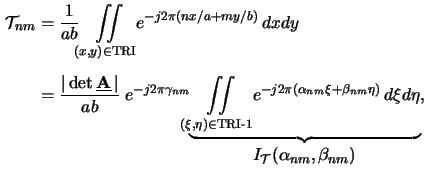whereby TRI-1 refers to the unit triangle and the coefficients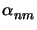,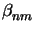and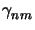are given by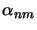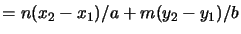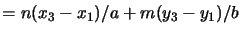(B.4)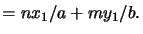The function I(,) is calculated as follows:a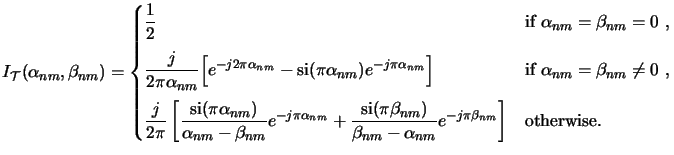(B.5)

#### Footnotes

... follows:a
The function si(x) is defined as si(x)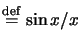.Next: B.2 Rectangular Patterns Up: B. Analytical Fourier Transformations Previous: B. Analytical Fourier Transformations
Heinrich Kirchauer, Institute for Microelectronics, TU Vienna
1998-04-17